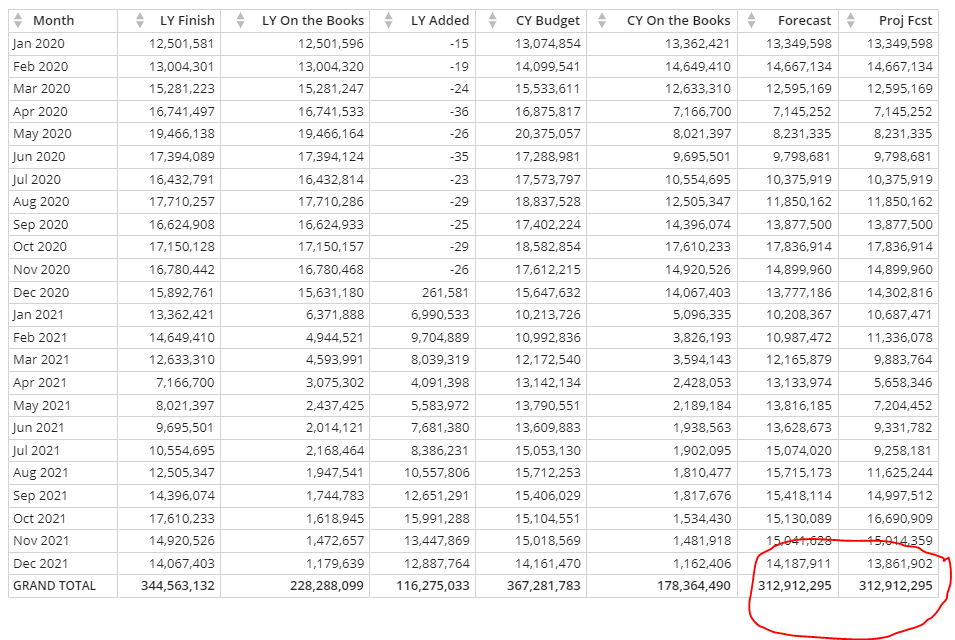# Beast Mode Case Statement Pivot Table issue

I have a Beast Mode calculation that has a case statement that looks to pull actual data from the past and projected data from the future.  Everything works fine in the pivot table card with the exception of the total.  It appears that the case statement is affecting the total instead of the pivot table just summing the individual values.  Is this a bug or expected behavior?  Any suggesting for solving or a work around would be much appreciated!

Below is the formula and the result.

(CASE
WHEN `IMPORT_CLOSE_DATE`<= CURRENT_DATE() then sum(`Pacing_Revenue_Forecast GROSS`)
ELSE (sum(`CY On the Books`)/ sum(`LY On the Books`)* SUM(`LY Finish`-`LY On the Books`))+ (sum(`CY On the Books`))
END)• Hi @user19682

This behavior is to be expected when using aggregate functions in beast modes. It's not a bug since it's actually beneficial for certain calculations, such as percentages or ratios, where adding up the individual values is not necessarily meaningful.

With that in mind, I would suggest using a dataflow to group all your data by month, then creating the same calculation for Proj Fcst but without using SUM functions. That way you can completely avoid using aggregate functions in a beast modes and let the total row use a simple sum rather than it applying the formula to your total as a whole.

• It's behaving as designed.

because your CASE statement is OUTSIDE of the SUM, it applies the CASE AFTER aggregation has happened, or to use your language "the case statement is affecting the total instead of the ... the individual values. "

To get your total to work as expected you have to put the CASE statement INSIDE of the aggregation.

SUM(CASE ...)